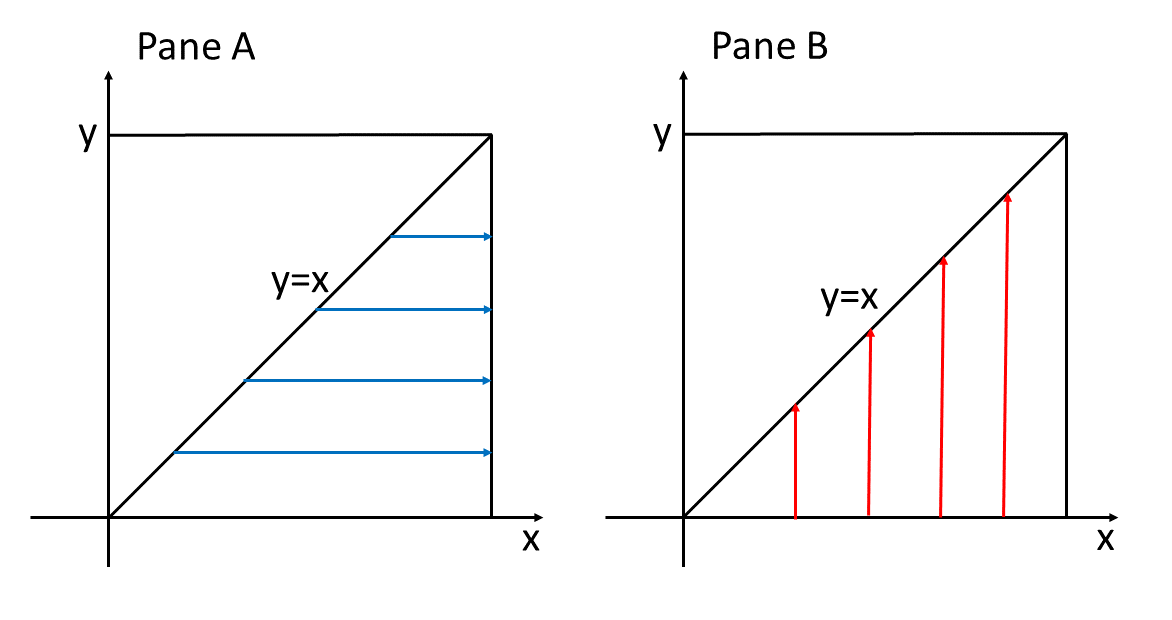24
Oct 22

## A problem to do once and never come back

There is a problem I gave on the midterm that does not require much imagination. Just know the definitions and do the technical work, so I was hoping we could put this behind us. Turned out we could not and thus you see this post.

Problem. Suppose the joint density of variables$X,Y$ is given by$f_{X,Y}(x,y)=\left\{ \begin{array}{c}k\left( e^{x}+e^{y}\right) \text{ for }0

I. Find$k$.

II. Find marginal densities of$X,Y$. Are$X,Y$ independent?

III. Find conditional densities$f_{X|Y},\ f_{Y|X}$.

IV. Find$EX,\ EY$.

When solving a problem like this, the first thing to do is to give the theory. You may not be able to finish without errors the long calculations but your grade will be determined by the beginning theoretical remarks.

### I. Finding the normalizing constant

Any density should satisfy the completeness axiom: the area under the density curve (or in this case the volume under the density surface) must be equal to one:$\int \int f_{X,Y}(x,y)dxdy=1.$ The constant$k$ chosen to satisfy this condition is called a normalizing constant. The integration in general is over the whole plain$R^{2}$ and the first task is to express the above integral as an iterated integral. This is where the domain where the density is not zero should be taken into account. There is little you can do without geometry. One example of how to do this is here.

The shape of the area$A=\left\{ (x,y):0 is determined by a) the extreme values of$x,y$ and b) the relationship between them. The extreme values are 0 and 1 for both$x$ and$y$, meaning that$A$ is contained in the square$\left\{ (x,y):0 The inequality$y means that we cut out of this square the triangle below the line$y=x$ (it is really the lower triangle because if from a point on the line$y=x$ we move down vertically,$x$ will stay the same and$y$ will become smaller than$x$).

In the iterated integral:

a) the lower and upper limits of integration for the inner integral are the boundaries for the inner variable; they may depend on the outer variable but not on the inner variable.

b) the lower and upper limits of integration for the outer integral are the extreme values for the outer variable; they must be constant.

This is illustrated in Pane A of Figure 1.Figure 1. Integration order

Always take the inner integral in parentheses to show that you are dealing with an iterated integral.

a) In the inner integral integrating over$x$ means moving along blue arrows from the boundary$x=y$ to the boundary$x=1.$ The boundaries may depend on$y$ but not on$x$ because the outer integral is over$y.$

b) In the outer integral put the extreme values for the outer variable. Thus,$\underset{A}{\int \int }f_{X,Y}(x,y)dxdy=\int_{0}^{1}\left(\int_{y}^{1}f_{X,Y}(x,y)dx\right) dy.$

Check that if we first integrate over$y$ (vertically along red arrows, see Pane B in Figure 1) then the equation$\underset{A}{\int \int }f_{X,Y}(x,y)dxdy=\int_{0}^{1}\left(\int_{0}^{x}f_{X,Y}(x,y)dy\right) dx$

results.

In fact, from the definition$A=\left\{ (x,y):0 one can see that the inner interval for$x$ is$\left[ y,1\right]$ and for$y$ it is$\left[ 0,x\right] .$

### II. Marginal densities

The condition for independence of$X,Y$ is$f_{X,Y}\left( x,y\right) =f_{X}\left( x\right) f_{Y}\left( y\right)$ (this is a direct analog of the independence condition for events$P\left( A\cap B\right) =P\left( A\right) P\left( B\right)$). In words: the joint density decomposes into a product of individual densities.

### III. Conditional densities

In this case the easiest is to recall the definition of conditional probability$P\left( A|B\right) =\frac{P\left( A\cap B\right) }{P\left(B\right) }.$ The definition of conditional densities$f_{X|Y},\ f_{Y|X}$ is quite similar:

(2)$f_{X|Y}\left( x|y\right) =\frac{f_{X,Y}\left( x,y\right) }{f_{Y}\left( y\right) },\ f_{Y|X}\left( y|x\right) =\frac{f_{X,Y}\left( x,y\right) }{f_{X}\left( x\right) }$.

Of course,$f_{Y}\left( y\right) ,f_{X}\left( x\right)$ here can be replaced by their marginal equivalents.

### IV. Finding expected values of$X,Y$$X,Y$

The usual definition$EX=\int xf_{X}\left( x\right) dx$ takes an equivalent form using the marginal density:$EX=\int x\left( \int f_{X,Y}\left( x,y\right) dy\right) dx=\int \int xf_{X,Y}\left( x,y\right) dydx.$

Which equation to use is a matter of convenience.

Another replacement in the usual definition gives the definition of conditional expectations:$E\left( X|Y\right) =\int xf_{X|Y}\left( x|y\right) dx,$$E\left( Y|X\right) =\int yf_{Y|X}\left( y|x\right) dx.$

Note that these are random variables:$E\left( X|Y=y\right)$ depends in$y$ and$E\left( Y|X=x\right)$ depends on$x.$

### Solution to the problem

Being a lazy guy, for the problem this post is about I provide answers found in Mathematica:

I.$k=0.581977$

II.$f_{X}\left( x\right) =-1+e^{x}\left( 1+x\right) ,$ for$x\in[ 0,1],$$f_{Y}\left( y\right) =e-e^{y}y,$ for$y\in \left[ 0,1\right] .$

It is readily seen that the independence condition is not satisfied.

III.$f_{X|Y}\left( x|y\right) =\frac{k\left( e^{x}+e^{y}\right) }{e-e^{y}y}$ for$0$f_{Y|X}\left(y|x\right) =\frac{k\left(e^x+e^y\right) }{-1+e^x\left( 1+x\right) }$ for$0

IV.$EX=0.709012,$$EY=0.372965.$